# Exploring interactions with continuous predictors in regression models

#### 2018-02-13

Understanding an interaction effect in a linear regression model is usually difficult when using just the basic output tables and looking at the coefficients. The jtools package provides several functions that can help analysts probe more deeply.

Categorical by categorical interactions: All the tools described here require at least one variable to be continuous. A separate vignette describes cat_plot, which handles the plotting of interactions in which all the focal predictors are categorical variables.

## Plotting interactions

First, we use example data from state.x77 that is built into R. Let’s look at the interaction model output with summ as a starting point.

fiti <- lm(Income ~ Illiteracy * Murder, data = as.data.frame(state.x77))
summ(fiti)
## MODEL INFO:
## Observations: 50
## Dependent Variable: Income
##
## MODEL FIT:
## F(3,46) = 7.46, p = 0
## R-squared = 0.33
##
## Standard errors: OLS
##                      Est.   S.E. t val.    p
## (Intercept)       3822.61 405.33   9.43 0    ***
## Illiteracy         617.34 434.85   1.42 0.16
## Murder             146.82  50.33   2.92 0.01  **
## Illiteracy:Murder -117.1   40.13  -2.92 0.01  **

So we see a significant main effect of Murder on Income in the presence of a significant interaction between Murder and Illiteracy. The positive estimate for Illiteracy does not differ significantly from zero. With that said, you shouldn’t focus too much on the main effects of terms included in the interaction.

Note that if you would like to see the output if the input variables were mean-centered and/or standardized, summ can do that for you.

summ(fiti, scale = TRUE)
## MODEL INFO:
## Observations: 50
## Dependent Variable: Income
##
## MODEL FIT:
## F(3,46) = 7.46, p = 0
## R-squared = 0.33
##
## Standard errors: OLS
##                      Est.   S.E. t val.    p
## (Intercept)       4617.31  96.34  47.93 0    ***
## Illiteracy        -150.31 122.06  -1.23 0.22
## Murder              36.23 106.32   0.34 0.73
## Illiteracy:Murder -263.48  90.3   -2.92 0.01  **
##
## All continuous predictors are mean-centered and scaled by 1 s.d.

A versatile and sometimes the most interpretable method for understanding interaction effects is via plotting. jtools provides interact_plot as a relatively pain-free method to get good-looking plots of interactions using ggplot2 on the backend.

interact_plot(fiti, pred = "Illiteracy", modx = "Murder")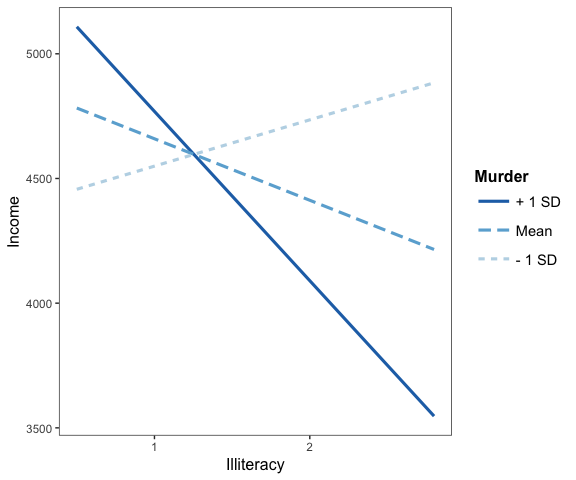Keep in mind that the default behavior of interact_plot is to mean-center all variables not involved in the interaction so that the predicted values are more easily interpreted. You can disable that by adding centered = "none".

By default, with a continuous moderator you get three lines—1 standard deviation above and below the mean and the mean itself. If you specify modxvals = "plus-minus", the mean of the moderator is not plotted, just the two +/- SD lines.

interact_plot(fiti, pred = "Illiteracy", modx = "Murder", modxvals = "plus-minus")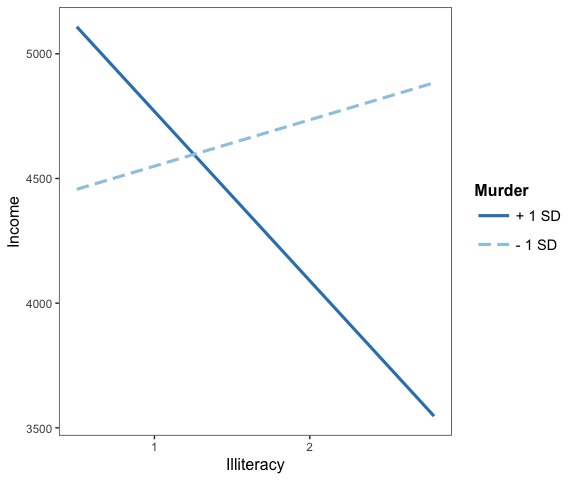However, if your moderator is a factor, each level will be plotted and you should leave modxvals = NULL, the default.

fitiris <- lm(Petal.Length ~ Petal.Width * Species, data = iris)
interact_plot(fitiris, pred = "Petal.Width", modx = "Species")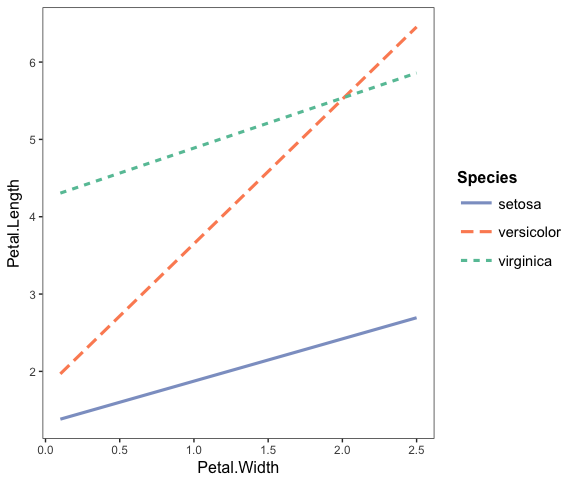### Plotting observed data

If you want to see the individual data points plotted to better understand how the fitted lines related to the observed data, you can use the plot.points = TRUE argument.

interact_plot(fiti, pred = "Illiteracy", modx = "Murder", plot.points = TRUE)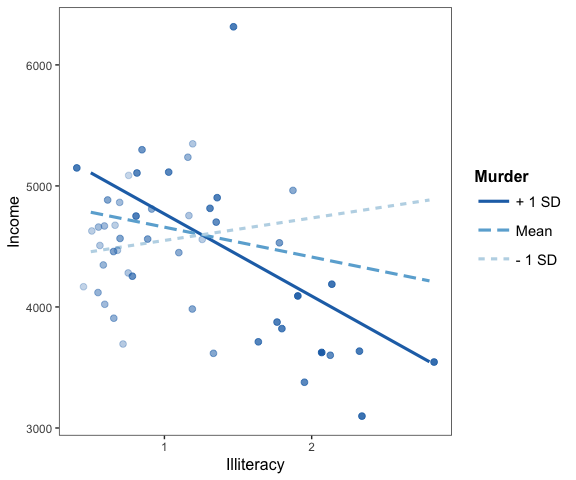For continuous moderators, as you can see, the observed data points are shaded depending on the level of the mdoerator variable.

It can be very enlightening, too, for categorical moderators.

interact_plot(fitiris, pred = "Petal.Width", modx = "Species", plot.points = TRUE)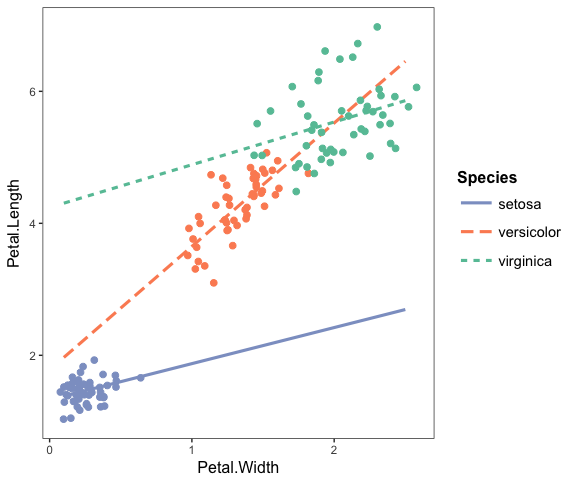If your original data are weighted, then the points will be sized based on the weight. For the purposes of our example, we’ll weight the same model we’ve been using with the population of each state.

fiti <- lm(Income ~ Illiteracy * Murder, data = as.data.frame(state.x77),
weights = Population)
interact_plot(fiti, pred = "Illiteracy", modx = "Murder", plot.points = TRUE)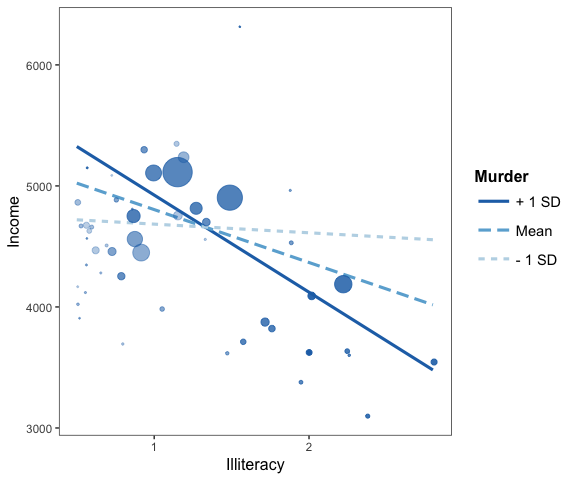For those working with weighted data, it can be hard to use a scatterplot to explore the data unless there is some way to account for the weights. Using size is a nice middle ground.

### Confidence bands

Another way to get a sense of the precision of the estimates is by plotting confidence bands. To get started, just set interval = TRUE. To decide how wide the confidence interval should be, express the percentile as a number, e.g., int.width = 0.8 corresponds to an 80% interval.

interact_plot(fiti, pred = "Illiteracy", modx = "Murder", interval = TRUE, int.width = 0.8)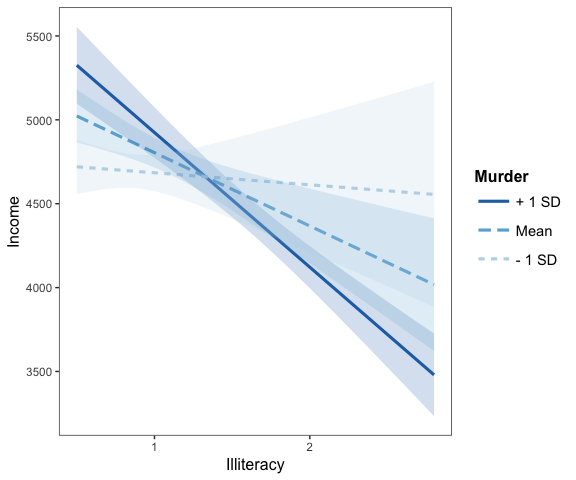### Other options

There are a number of other options not mentioned, many relating to the appearance.

For instance, you can manually specify the axis labels, add a main title, choose a color scheme, and so on.

interact_plot(fiti, pred = "Illiteracy", modx = "Murder",
x.label = "Custom X Label", y.label = "Custom Y Label",
main.title = "Sample Plot",  legend.main = "Custom Legend Title",
color.class = "Oranges")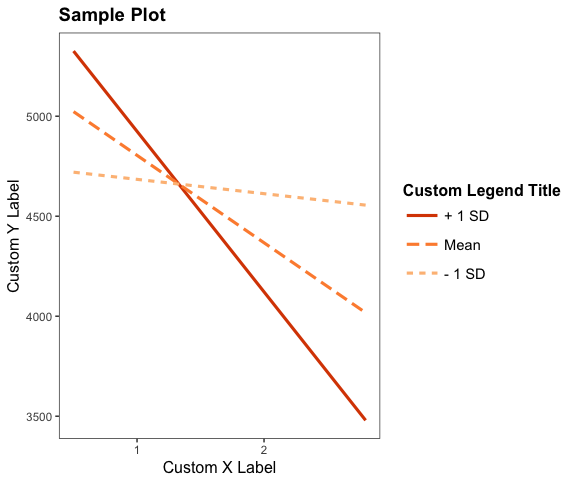Because the function uses ggplot2, it can be modified and extended like any other ggplot2 object. For example, using the theme_apa function from jtools:

interact_plot(fitiris, pred = "Petal.Width", modx = "Species") + theme_apa()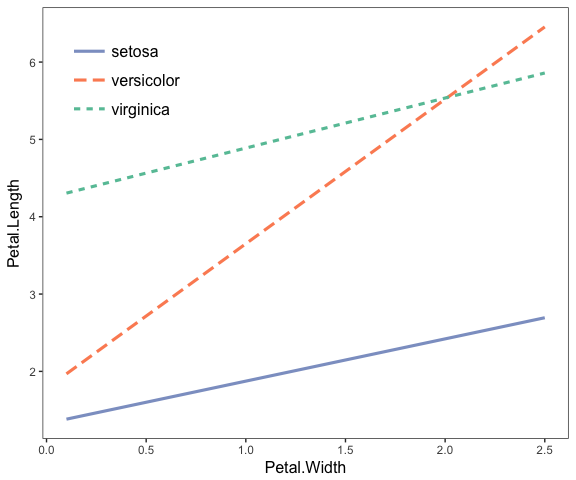## Simple slopes analysis and Johnson-Neyman intervals

Simple slopes analysis gives researchers a way to express the interaction effect in terms that are easy to understand to those who know how to interpret direct effects in regression models. This method is designed for continuous variable by continuous variable interactions, but can work when the moderator is binary.

In simple slopes analysis, researchers are interested in the conditional slope of the focal predictor; that is, what is the slope of the predictor when the moderator is held at some particular value? The regression output we get when including the interaction term tells us what the slope is when the moderator is held at zero, which is often not a practically/theoretically meaningful value. To better understand the nature of the interaction, simple slopes analysis allows the researcher to specify meaningful values at which to hold the moderator value.

While the computation behind doing so isn’t exactly rocket science, it is inconvenient and prone to error. The sim_slopes function from jtools accepts a regression model (with an interaction term) as an input and automates the simple slopes procedure. The function will, by default, do the following:

• Mean-center all non-focal predictors (so that setting them to zero means setting them to their mean)
• For continuous moderators, it will choose the mean as well as the mean plus/minus 1 standard deviation as values at which to find the slope of the focal predictor.
• For categorical/binary moderators, it will find the slope of the focal predictor at each level of the moderator.

In its most basic use case, sim_slopes needs three arguments: a linear model (with support for svyglm models), the name of the focal predictor in quotations as the argument for pred =, and the name of the moderator in quotations as the argument for modx =. Let’s go through an example.

Don’t sweat the change in the significance of the lower-order focal variables—they aren’t directly interpretable, anyway.

Now let’s do the most basic simple slopes analysis:

sim_slopes(fiti, pred = Illiteracy, modx = Murder, johnson_neyman = FALSE)
## SIMPLE SLOPES ANALYSIS
##
## Slope of Illiteracy when Murder = 11.95 (+ 1 SD):
##    Est.    S.E.       p
## -802.66  145.72    0.00
##
## Slope of Illiteracy when Murder = 8.69 (Mean):
##    Est.    S.E.       p
## -437.12  175.82    0.02
##
## Slope of Illiteracy when Murder = 5.42 (- 1 SD):
##   Est.   S.E.      p
## -71.59 268.65   0.79

So what we see in this example is that when the value of Murder is high, the slope of Illiteracy is negative and significantly different from zero. The value for Illiteracy when Murder is high is in the opposite direction from its coefficient estimate for the first version of the model fit with lm but this result makes sense considering the interaction coefficient was negative; it means that as one of the variables goes up, the other goes down. Now we know the effect of Illiteracy only exists when Murder is high.

You may also choose the values of the moderator yourself with the modxvals = argument.

sim_slopes(fiti, pred = Illiteracy, modx = Murder, modxvals = c(0, 5, 10),
johnson_neyman = FALSE)
## SIMPLE SLOPES ANALYSIS
##
## Slope of Illiteracy when Murder = 10:
##    Est.    S.E.       p
## -584.38  152.37    0.00
##
## Slope of Illiteracy when Murder = 5:
##   Est.   S.E.      p
## -24.44 282.48   0.93
##
## Slope of Illiteracy when Murder = 0:
##   Est.   S.E.      p
## 535.50 458.77   0.25

### Johnson-Neyman intervals

Did you notice how I was adding the argument johnson_neyman = FALSE above? That’s because by default, sim_slopes will also calculate what is called the Johnson-Neyman interval. This tells you all the values of the moderator for which the slope of the predictor will be statistically significant. Depending on the specific analysis, it may be that all values of the moderator outside of the interval will have a significant slope for the predictor. Other times, it will only be values inside the interval—you will have to look at the output to see.

It can take a moment to interpret this correctly if you aren’t familiar with the Johnson-Neyman technique. But if you read the output carefully and take it literally, you’ll get the hang of it.

sim_slopes(fiti, pred = Illiteracy, modx = Murder, johnson_neyman = TRUE)
## JOHNSON-NEYMAN INTERVAL
##
## The slope of Illiteracy is p < .05 when Murder is OUTSIDE this interval:
## [-10.61, 8.16]
## Note: The range of observed values of Murder is [1.4, 15.1]
##
## SIMPLE SLOPES ANALYSIS
##
## Slope of Illiteracy when Murder = 11.95 (+ 1 SD):
##    Est.    S.E.       p
## -802.66  145.72    0.00
##
## Slope of Illiteracy when Murder = 8.69 (Mean):
##    Est.    S.E.       p
## -437.12  175.82    0.02
##
## Slope of Illiteracy when Murder = 5.42 (- 1 SD):
##   Est.   S.E.      p
## -71.59 268.65   0.79

So in the example above, we can see that the Johnson-Neyman interval and the simple slopes analysis agree—they always will. The benefit of the J-N interval is it will tell you exactly where the predictor’s slope becomes significant/insignificant. You can also call the johnson_neyman function directly if you want to do something like tweak the alpha level. The johnson_neyman function will also create a plot by default—you can get them by setting jnplot = TRUE with sim_slopes.

johnson_neyman(fiti, pred = Illiteracy, modx = Murder, alpha = 0.01)
## JOHNSON-NEYMAN INTERVAL
##
## The slope of Illiteracy is p < .01 when Murder is OUTSIDE this interval:
## [-75.31, 8.89]
## Note: The range of observed values of Murder is [1.4, 15.1]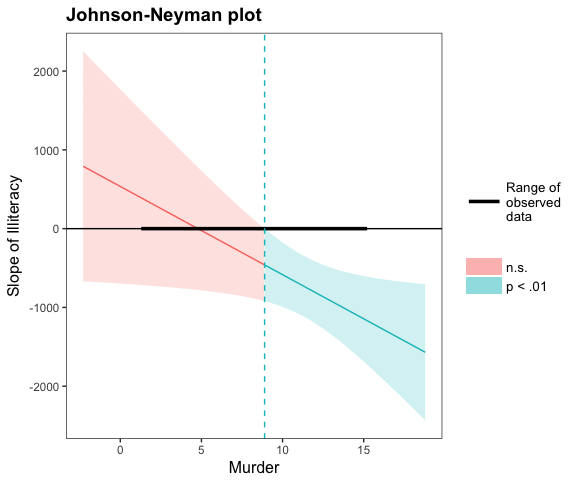One note on Johnson-Neyman plots: Once again, it is easy to misinterpret the meaning. Notice that the y-axis is the conditional slope of the predictor. The plot shows you where the conditional slope differs significantly from zero. In the plot above, we see that from the point Murder (the moderator) = 9.12 and greater, the slope of Illiteracy (the predictor) is significantly different from zero and in this case negative. The lower bound for this interval (about -32) is so far outside the observed data that it is not plotted. If you could have -32 as a value for Murder rate, though, that would be the other threshold before which the slope of Illiteracy would become positive.

The purpose of reminding you both within the plot and the printed output of the range of observed data is to help you put the results in context; in this case, the only justifiable interpretation is that Illiteracy has no effect on the outcome variable except when Murder is higher than 9.12. You wouldn’t interpret the lower boundary because your dataset doesn’t contain any values near it.

A recent publication (Esarey & Sumner, 2017) explored ways to calculate the Johnson-Neyman interval that properly manages the Type I and II error rates. Others have noted that the alpha level implied by the Johnson-Neyman interval won’t be quite right (e.g., Bauer & Curran, 2005), but there hasn’t been any general solution that has gotten wide acceptance in the research literature just yet.

The basic problem is that the Johnson-Neyman interval is essentially doing a bunch of comparisons across all the values of the moderator, each one inflating the Type I error rate. The issue isn’t so much that you can’t possibly address it, but many solutions are far too conservative and others aren’t broadly applicable. Esarey and Sumner (2017), among other contributions, suggested an adjustment that seems to do a good job of balancing the desire to be a conservative test without missing a lot of true effects in the process. I won’t go into the details here. The implementation in johnson_neyman is based on code adapted from Esarey and Sumner’s interactionTest package, but any errors should be assumed to be from jtools, not them.

To use this feature, simply set control.fdr = TRUE in the call to johnson_neyman or sim_slopes.

sim_slopes(fiti, pred = Illiteracy, modx = Murder, johnson_neyman = TRUE,
control.fdr = TRUE)
## JOHNSON-NEYMAN INTERVAL
##
## The slope of Illiteracy is p < .05 when Murder is OUTSIDE this interval:
## [-22.57, 8.52]
## Note: The range of observed values of Murder is [1.4, 15.1]
##
## Interval calculated using false discovery rate adjusted t = 2.33
##
## SIMPLE SLOPES ANALYSIS
##
## Slope of Illiteracy when Murder = 11.95 (+ 1 SD):
##    Est.    S.E.       p
## -802.66  145.72    0.00
##
## Slope of Illiteracy when Murder = 8.69 (Mean):
##    Est.    S.E.       p
## -437.12  175.82    0.02
##
## Slope of Illiteracy when Murder = 5.42 (- 1 SD):
##   Est.   S.E.      p
## -71.59 268.65   0.79

In this case, you can see that the interval is just a little bit wider. The output reports the adjusted test statistic, which is 2.33, not much different than the (approximately) 2 that would be used otherwise. In other cases it may be quite a bit larger.

#### Conditional intercepts

Sometimes it is informative to know the conditional intercepts in addition to the slopes. It might be interesting to you that individuals low on the moderator have a positive slope and individuals high on it don’t, but that doesn’t mean that individuals low on the moderator will have higher values of the dependent variable. You would only know that if you know the conditional intercept.

You can print the conditional intercepts with the cond.int = TRUE argument.

sim_slopes(fiti, pred = Illiteracy, modx = Murder, cond.int = TRUE)
## JOHNSON-NEYMAN INTERVAL
##
## The slope of Illiteracy is p < .05 when Murder is OUTSIDE this interval:
## [-10.61, 8.16]
## Note: The range of observed values of Murder is [1.4, 15.1]
##
## SIMPLE SLOPES ANALYSIS
##
## Slope of Illiteracy when Murder = 11.95 (+ 1 SD):
##    Est.    S.E.       p
## -802.66  145.72    0.00
## Conditional intercept when Murder = 11.95 (+ 1 SD):
##    Est.    S.E.       p
## 5726.98  246.24    0.00
##
## Slope of Illiteracy when Murder = 8.69 (Mean):
##    Est.    S.E.       p
## -437.12  175.82    0.02
## Conditional intercept when Murder = 8.69 (Mean):
##    Est.    S.E.       p
## 5241.43  202.73    0.00
##
## Slope of Illiteracy when Murder = 5.42 (- 1 SD):
##   Est.   S.E.      p
## -71.59 268.65   0.79
## Conditional intercept when Murder = 5.42 (- 1 SD):
##    Est.    S.E.       p
## 4755.88  247.48    0.00

This example shows you that while the slope associated with Illiteracy is negative when Murder is high, the conditional intercept is also high when Murder is high. That tells you that increases in Illiteracy for high-Murder observations will tend towards being equal on Income to observations with lower values of Murder.

#### Robust standard errors

Certain models require heteroskedasticity-robust standard errors. To be consistent with the reporting of heteroskedasticity-robust standard errors offered by summ, sim_slopes will do the same with the use of the robust = TRUE option so you can consistently report standard errors across models.

sim_slopes(fiti, pred = Illiteracy, modx = Murder, robust = TRUE)
## JOHNSON-NEYMAN INTERVAL
##
## The slope of Illiteracy is p < .05 when Murder is OUTSIDE this interval:
## [-6.13, 8.31]
## Note: The range of observed values of Murder is [1.4, 15.1]
##
## SIMPLE SLOPES ANALYSIS
##
## Slope of Illiteracy when Murder = 11.95 (+ 1 SD):
##    Est.    S.E.       p
## -802.66  178.75    0.00
##
## Slope of Illiteracy when Murder = 8.69 (Mean):
##    Est.    S.E.       p
## -437.12  191.07    0.03
##
## Slope of Illiteracy when Murder = 5.42 (- 1 SD):
##   Est.   S.E.      p
## -71.59 256.60   0.78

These data are a relatively rare case in which the robust standard errors are even more efficient than typical OLS standard errors. Note that you must have the sandwich package installed to use this feature.

#### Centering and standardizing variables

By default, all non-focal variables are mean-centered. You can additionally specify that the focal predictors are centered with centered = "all". You may also request that no variables be centered with centered = "none". You may also request specific variables to center by providing a vector of quoted variable names—no others will be centered in that case.

Note that the moderator is centered around the specified values. Factor variables are ignored in the centering process.

You can standardize the variables as well using the scale = TRUE argument.

sim_slopes(fiti, pred = Illiteracy, modx = Murder, scale = TRUE,
centered = "all")
## JOHNSON-NEYMAN INTERVAL
##
## The slope of Illiteracy is p < .05 when Murder is OUTSIDE this interval:
## [-5.91, -0.16]
## Note: The range of observed values of Murder is [-2.23, 1.97]
##
## SIMPLE SLOPES ANALYSIS
##
## Slope of Illiteracy when Murder = 1 (+ 1 SD):
##    Est.    S.E.       p
## -427.07   77.53    0.00
##
## Slope of Illiteracy when Murder = 0 (Mean):
##    Est.    S.E.       p
## -232.58   93.55    0.02
##
## Slope of Illiteracy when Murder = -1 (- 1 SD):
##   Est.   S.E.      p
## -38.09 142.94   0.79

Standardization is only meaningful if applied to the focal predictors. If you use scale = TRUE when centered = NULL, the output won’t change since the scale of the other predictors has no direct effect on the others.

## Do simple slopes and plotting with one function

You won’t have to use these functions long before you may find yourself using both of them for each model you want to explore. To streamline the process, this package offers probe_interaction() as a convenience function that calls both sim_slopes() and interact_plot(), taking advantage of their overlapping syntax.

library(survey)
data(api)
dstrat <- svydesign(id = ~1, strata = ~stype, weights = ~pw,
data = apistrat, fpc = ~fpc)
regmodel <- svyglm(api00 ~ avg.ed * growth, design = dstrat)

probe_interaction(regmodel, pred = growth, modx = avg.ed, cond.int = TRUE,
interval = TRUE,  jnplot = TRUE)
## JOHNSON-NEYMAN INTERVAL
##
## The slope of growth is p < .05 when avg.ed is OUTSIDE this interval:
## [3.11, 5.78]
## Note: The range of observed values of avg.ed is [1.38, 4.44]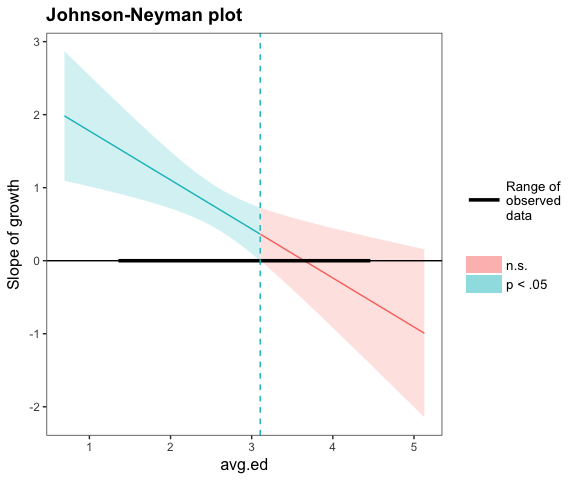## SIMPLE SLOPES ANALYSIS
##
## Slope of growth when avg.ed = 3.49 (+ 1 SD):
## Est. S.E.    p
## 0.11 0.25 0.67
## Conditional intercept when avg.ed = 3.49 (+ 1 SD):
##   Est.   S.E.      p
## 758.34   6.85   0.00
##
## Slope of growth when avg.ed = 2.79 (Mean):
## Est. S.E.    p
## 0.58 0.15 0.00
## Conditional intercept when avg.ed = 2.79 (Mean):
##   Est.   S.E.      p
## 639.78   6.80   0.00
##
## Slope of growth when avg.ed = 2.09 (- 1 SD):
## Est. S.E.    p
## 1.05 0.19 0.00
## Conditional intercept when avg.ed = 2.09 (- 1 SD):
##   Est.   S.E.      p
## 521.23  11.20   0.00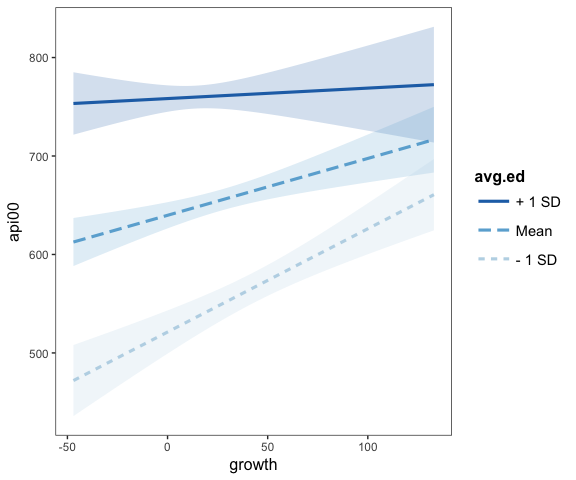Note in the above example that you can provide arguments that only apply to one function and they will be used appropriately. On the other hand, you cannot apply their overlapping functions selectively. That is, you can’t have one scale = TRUE and the other scale = FALSE. If you want that level of control, just call each function separately.

Also, the above example comes from the survey package as a means to show that, yes, these tools can be used with svyglm objects, though should only be applied to linear models.

It returns an object with each of the two functions’ return objects:

out <- probe_interaction(regmodel, pred = growth, modx = avg.ed,
cond.int = TRUE, interval = TRUE, jnplot = TRUE)
names(out)
##  "simslopes"    "interactplot"

## 3-way interactions

If 2-way interactions can be hard to grasp by looking at regular regression output, then 3-way interactions are outright inscrutable. The aforementioned functions also support 3-way interactions, however. Plotting these effects is particularly helpful.

Note that Johnson-Neyman intervals are still provided, but only insofar as you get the intervals for chosen levels of the second moderator. This does go against some of the distinctiveness of the J-N technique, which for 2-way interactions is a way to avoid having to choose points of the moderator to check whether the predictor has a significant slope.

fita3 <- lm(rating ~ privileges * critical * learning, data = attitude)
probe_interaction(fita3, pred = critical, modx = learning, mod2 = privileges)
## ############################################################
## While privileges (2nd moderator) = 40.9 (Mean of privileges - 1 SD)
## ############################################################
##
## JOHNSON-NEYMAN INTERVAL
##
## The Johnson-Neyman interval could not be found.
## Is your interaction term significant?
##
## SIMPLE SLOPES ANALYSIS
##
## Slope of critical when learning = 44.63 (- 1 SD):
## Est. S.E.    p
## 0.24 0.24 0.32
##
## Slope of critical when learning = 56.37 (Mean):
## Est. S.E.    p
## 0.68 0.33 0.05
##
## Slope of critical when learning = 68.1 (+ 1 SD):
## Est. S.E.    p
## 1.11 0.55 0.06
##
## ############################################################
## While privileges (2nd moderator) = 53.13 (Mean of privileges)
## ############################################################
##
## JOHNSON-NEYMAN INTERVAL
##
## The Johnson-Neyman interval could not be found.
## Is your interaction term significant?
##
## SIMPLE SLOPES ANALYSIS
##
## Slope of critical when learning = 44.63 (- 1 SD):
## Est. S.E.    p
## 0.09 0.34 0.79
##
## Slope of critical when learning = 56.37 (Mean):
## Est. S.E.    p
## 0.06 0.24 0.81
##
## Slope of critical when learning = 68.1 (+ 1 SD):
## Est. S.E.    p
## 0.02 0.33 0.95
##
## ############################################################
## While privileges (2nd moderator) = 65.37 (Mean of privileges + 1 SD)
## ############################################################
##
## JOHNSON-NEYMAN INTERVAL
##
## The Johnson-Neyman interval could not be found.
## Is your interaction term significant?
##
## SIMPLE SLOPES ANALYSIS
##
## Slope of critical when learning = 44.63 (- 1 SD):
##  Est.  S.E.     p
## -0.06  0.61  0.93
##
## Slope of critical when learning = 56.37 (Mean):
##  Est.  S.E.     p
## -0.56  0.50  0.28
##
## Slope of critical when learning = 68.1 (+ 1 SD):
##  Est.  S.E.     p
## -1.06  0.66  0.12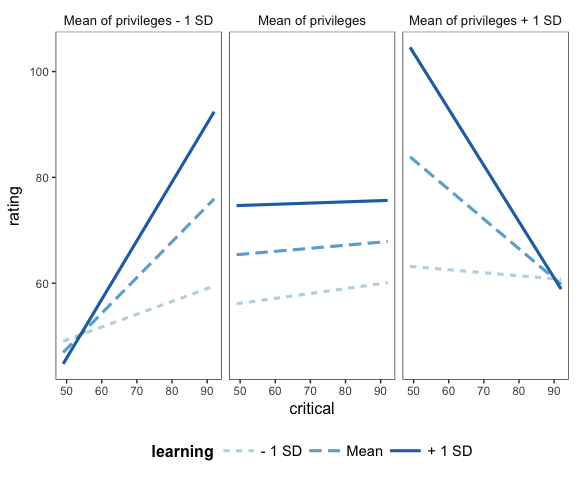You can change the labels for each plot via the mod2.labels argument.

interact_plot has a bit more flexibility here than sim_slopes, allowing for factor moderators with greater than 2 levels. And don’t forget that you can use theme_apa to format for publications or just to make more economical use of space.

mtcars$cyl <- factor(mtcars$cyl,
labels = c("4 cylinder", "6 cylinder", "8 cylinder"))
fitc3 <- lm(mpg ~ hp * wt * cyl, data = mtcars)
interact_plot(fitc3, pred = hp, modx = wt, mod2 = cyl) +
theme_apa(legend.pos = "bottomright")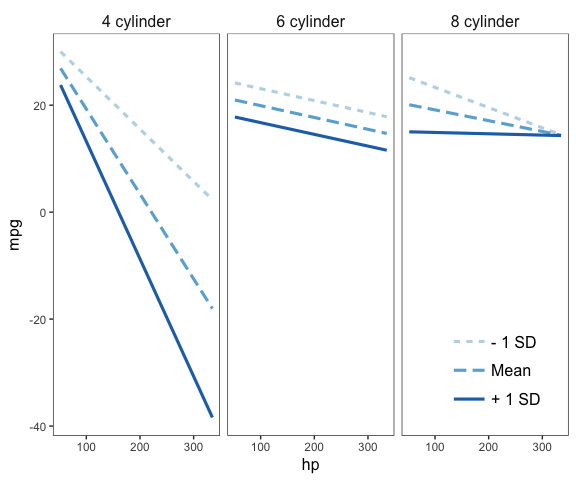You can get Johnson-Neyman plots for 3-way interactions as well, but keep in mind what I mentioned earlier in this section about the J-N technique for 3-way interactions. You will also need the cowplot package, which is used on the backend to mush together the separate J-N plots.

regmodel3 <- svyglm(api00 ~ avg.ed * growth * enroll, design = dstrat)
sim_slopes(regmodel3, pred = growth, modx = avg.ed, mod2 = enroll,
jnplot = TRUE)
## ############################################################
## While enroll (2nd moderator) = 153.05 (Mean of enroll - 1 SD)
## ############################################################
##
## JOHNSON-NEYMAN INTERVAL
##
## The slope of growth is p < .05 when avg.ed is OUTSIDE this interval:
## [2.75, 3.82]
## Note: The range of observed values of avg.ed is [1.38, 4.44]
##
## SIMPLE SLOPES ANALYSIS
##
## Slope of growth when avg.ed = 2.09 (- 1 SD):
## Est. S.E.    p
## 1.25 0.32 0.00
##
## Slope of growth when avg.ed = 2.79 (Mean):
## Est. S.E.    p
## 0.39 0.22 0.08
##
## Slope of growth when avg.ed = 3.49 (+ 1 SD):
##  Est.  S.E.     p
## -0.48  0.35  0.17
##
## ############################################################
## While enroll (2nd moderator) = 595.28 (Mean of enroll)
## ############################################################
##
## JOHNSON-NEYMAN INTERVAL
##
## The slope of growth is p < .05 when avg.ed is OUTSIDE this interval:
## [2.84, 7.83]
## Note: The range of observed values of avg.ed is [1.38, 4.44]
##
## SIMPLE SLOPES ANALYSIS
##
## Slope of growth when avg.ed = 2.09 (- 1 SD):
## Est. S.E.    p
## 0.72 0.22 0.00
##
## Slope of growth when avg.ed = 2.79 (Mean):
## Est. S.E.    p
## 0.34 0.16 0.03
##
## Slope of growth when avg.ed = 3.49 (+ 1 SD):
##  Est.  S.E.     p
## -0.04  0.24  0.87
##
## ############################################################
## While enroll (2nd moderator) = 1037.51 (Mean of enroll + 1 SD)
## ############################################################
##
## JOHNSON-NEYMAN INTERVAL
##
## The Johnson-Neyman interval could not be found.
## Is your interaction term significant?
##
## SIMPLE SLOPES ANALYSIS
##
## Slope of growth when avg.ed = 2.09 (- 1 SD):
## Est. S.E.    p
## 0.18 0.31 0.56
##
## Slope of growth when avg.ed = 2.79 (Mean):
## Est. S.E.    p
## 0.29 0.20 0.14
##
## Slope of growth when avg.ed = 3.49 (+ 1 SD):
## Est. S.E.    p
## 0.40 0.27 0.14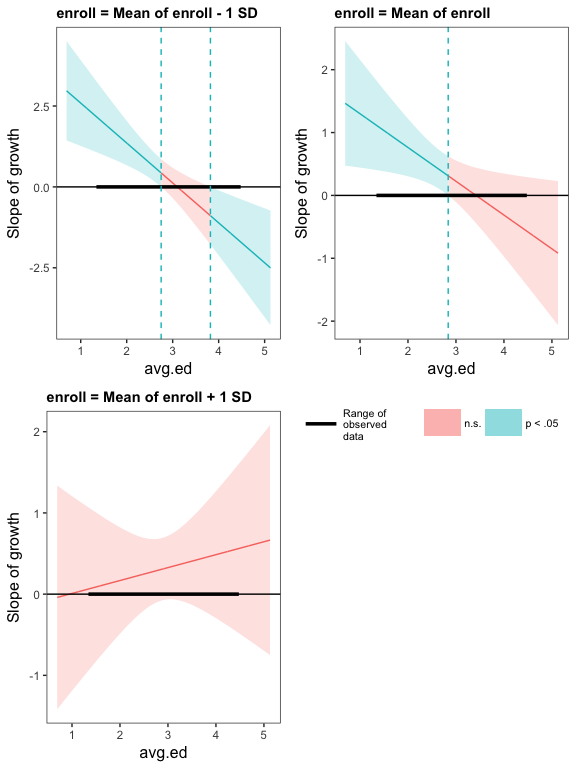Notice that at one of the three values of the second moderator, there were no Johnson-Neyman interval values so it wasn’t plotted. The more levels of the second moderator you want to plot, the more likely that the resulting plot will be unwieldy and hard to read. You can resize your window to help, though.

## Generalized linear models, mixed models, et al.

interact_plot is designed to be as general as possible and has been tested with glm, svyglm, and merMod models. When dealing with generalized linear models, it can be immensely useful to get a look at the predicted values on their response scale (e.g., the probability scale for logit models).

To give an example of how such a plot might look, I’ll generate some example data.

set.seed(5)
x <- rnorm(100)
m <- rnorm(100)
prob <- boot::inv.logit(.25 + .3*x + .3*m + -.5*(x*m) + rnorm(100))
y <- rep(0, 100)
y[prob >= .5] <- 1
logit_fit <- glm(y ~ x * m, family = binomial)

Here’s some summary output, for reference:

summ(logit_fit)
## MODEL INFO:
## Observations: 100
## Dependent Variable: y
## Error Distribution: binomial
##
## MODEL FIT:
## Pseudo R-squared (Cragg-Uhler) = 0.39
## Pseudo R-squared (McFadden) = 0.25
## AIC = 109.38, BIC = 119.81
##
## Standard errors: MLE
##              Est. S.E. z val.    p
## (Intercept)  0.58 0.25   2.27 0.02   *
## x            0.54 0.32   1.67 0.1    .
## m            0.86 0.33   2.62 0.01  **
## x:m         -1.73 0.46  -3.79 0    ***

Now let’s plot our logit model’s interaction:

interact_plot(logit_fit, pred = x, modx = m)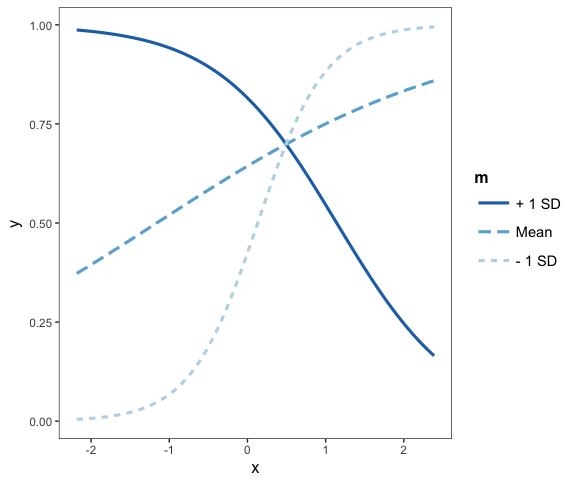A beautiful transverse interaction with the familiar logistic curve.